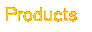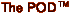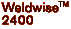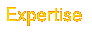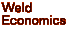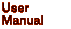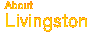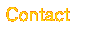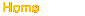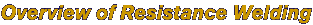Historical Background Introduction Variables in the Welding Process Critical Factors in Welding Electrodes, Surface Contact and Current Density Ohm's and Joule's Laws ------------------ Identifying and Correcting Weld Problems Industry Resource Addresses Right click here to download a complete PDF of the Rev.C Livingston POD User's Manual.
 Ohm's Law and Joule's Laws. The following laws are widely thought to be what make or break resistance welding. While it is true that these laws are very important to resistance welding, there are a few details that should be clarified. Ohm's Law states that V (Voltage) = I (Current) x R (Resistance). What does this mean in real-world terms? Returning to the pipe example, the more water pressure there is in a pipe (more voltage), the more water can flow through that pipe (more current). If the size of the pipe decreases (more resistance), then the water flow will decrease (less current) but the pressure drop along the pipe will increase (more voltage). Joule's Law states that H (Heat) = I (Current) x V (Voltage) x T (Time the current is allowed to flow). Or, written differently, H (Heat) = I2 (Current squared) x R (Resistance) x T (Time the current is allowed to flow). Note: V (Voltage) = I (Current) x R (Resistance), so the two equations are the same, just stated differently. The second version of this law is probably more common in the field. Joule's Law is an equation that gives the amount of heat (energy) delivered to something. It would seem sensible to assume that it's the amount of heat delivered to the weld. However, it is important to consider all the factors in the equation: Current, Voltage, and Time. Joule's Law assumes that each of these factors remains constant in the secondary of the welding transformer. A weld controller or weld timer may indeed provide a constant amount of current at the electrodes, but recall Ohm's Law: Voltage equals Current times Resistance, or written differently, Current equals Voltage divided by Resistance. Factors like pitting or mushrooming of the electrodes, dirty workpieces, changes in force, etc. all have an effect on the surface area (the area of contact) between the electrode and the workpiece. Since changes in the surface area affect the contact resistance (resistance of the surface area), it is reasonable to say that the resistance at the workpiece is not constant, but rather a factor that can change depending on a number of other conditions. If Resistance is not constant, then according to Ohm's Law, Current is not constant either. This means that the I-squared version Joule's Law will not reveal the amount of heat generated at the workpiece unless the resistance at the tips is known. Simply put, to determine how much heat is being generated at the workpiece using Joule's Law, current, voltage or resistance must be measured at the workpiece. Although a weld controller may be programmed to deliver 20 KA at 10 Volts, if there is significant resistance in the secondary weld loop, the heat will go there and not to the workpiece. Likewise, if the electrodes are worn or the workpiece is dirty, resistance and current density will be affected. In such a situation, a controller might indicate 10 Volts at the secondary, however there might actually be only 5 Volts at the weld tips. Such a disparity could easily cause bad welds.